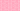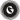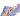PricingResources

# How to Calculate Standard DeviationWritten by

In finance, as in business, you need to establish a comfortable relationship with risk. As important as it is to be risk-averse, there’s always an element of risk in both business and investment. Risk isn’t always necessarily a bad thing. Risks can yield high returns in the world of investment. Likewise, a risky venture like branching into a new market or releasing a new product can help to take your business to the next level.

This is why risk management is a crucial discipline for investors and entrepreneurs alike to learn. Although nobody has a crystal ball, some mathematical concepts can help with managing risk and informing investment or operational strategies. Standard deviation is one such concept that can be a useful metric for measuring risk.

## What does standard deviation mean?

Standard deviation is a mathematical tool that’s often used by investors and economists to see how variable the flow of data (like the fluctuating price of a certain stock) is. Essentially, it measures how widely a data set is dispersed relative to its mean. So, in terms of the stock market, it might measure how often a stock drops and rises above its mean average range.

The further different data points are from the mean, the greater the standard deviation. From this, an investor may determine the risk-to-return ratio of that stock. High standard deviations tend to imply a volatile stock, while low standard deviations indicate that a stock is likely to be relatively stable.

## How to calculate standard deviation

Standard deviation is expressed as a square root of a variance. The formula for calculating standard deviation is as follows:

In this equation:

• r i is a single return observed within a data set

• r avg is the mean average of returns observed within a timeframe

• n is the number of observations included in the data set

So, to calculate the standard deviation on a stock, we need to:

• Calculate the mean value by adding all the data points together and dividing this by the number of data points.

• Determine the variance for each data point by subtracting the mean from the value of each data point. The resulting values are then squared and the results added up. The resulting figure is then divided by the number of data points minus one.

• The standard deviation is expressed as the square root of the variance calculated above.

## Standard deviation and the normal distribution of returns

Investment is all about the long game. The theory of normal distribution of returns stipulates that returns on any investment will fall somewhere on a bell-shaped curve. This bell curve is intrinsically tied to the principle of standard deviation.

On this bell curve:

• 68% of returns fall within 1 standard deviation of the mean average

• 95% of returns will fall within 2 standard deviations of the mean average

• 99% of returns will fall within 3 standard deviations of the mean average

## Why is standard deviation important?

Standard deviation is an important principle to understand. Especially if, like many self-employed sole traders and business owners, you rely on investments to fund your retirement in lieu of a conventional workplace pension.

Understanding standard deviation can help you to diversify your stock portfolio to provide a good balance of risk and reward. A lower standard deviation doesn’t necessarily mean a better investment. But it can be an indicator of predictability. Adjusting your ratios of safe and volatile stocks can help you to adjust your portfolio to achieve your long and short-term goals.

#### We can help

If you’re interested in finding out more about standard deviation, risk management, or any other aspect of your business finances, then get in touch with our financial experts. Find out how GoCardless can help you with ad hoc payments or recurring payments.Contact sales

Sales

Support